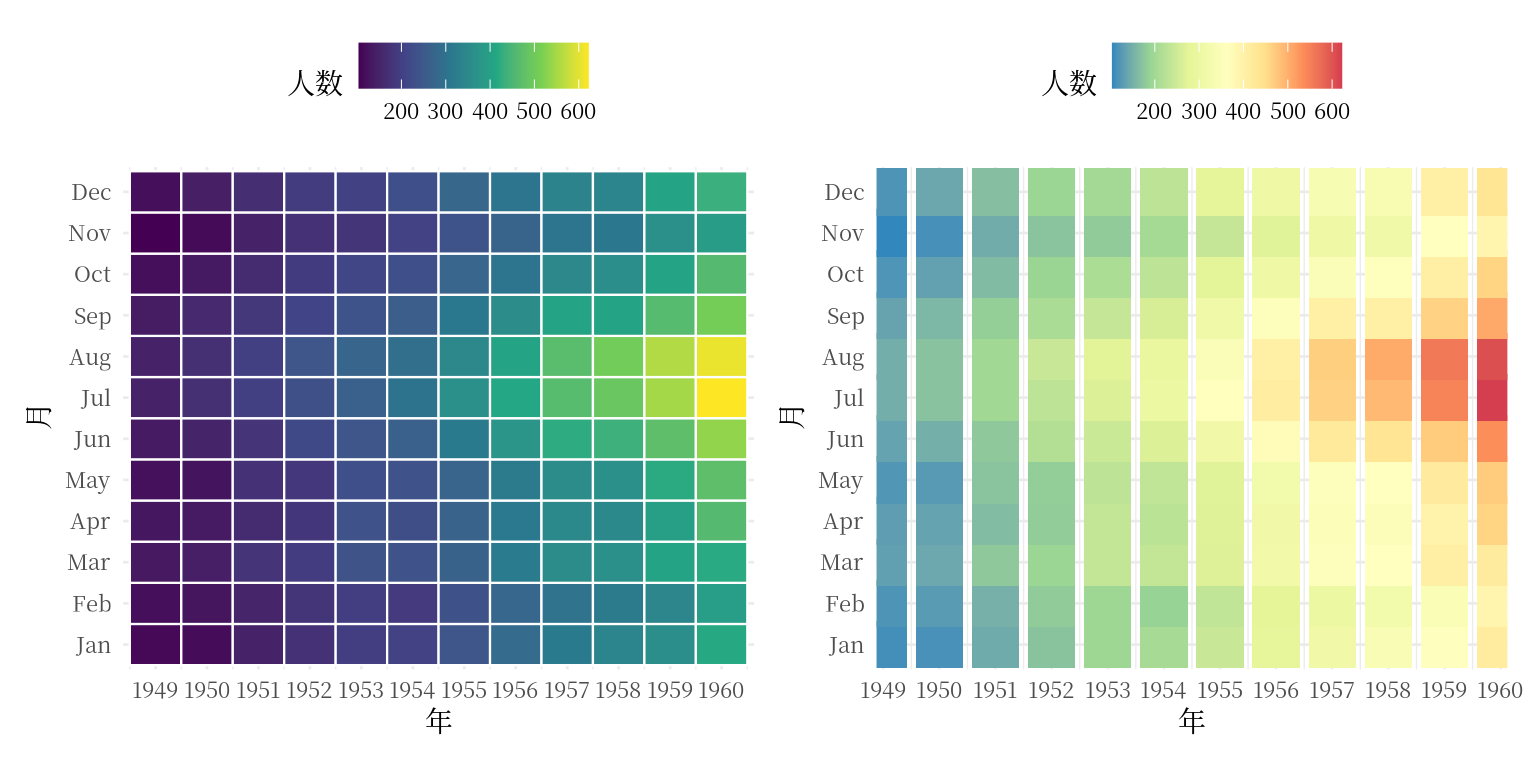## 12.14 瓦片图

p1 <- expand.grid(months = month.abb, years = 1949:1960) %>%
transform(num = as.vector(AirPassengers)) %>%
ggplot(aes(x = years, y = months, fill = num)) +
scale_fill_continuous(type = "viridis") +
geom_tile(color = "white", size = 0.4) +
scale_x_continuous(
expand = c(0.01, 0.01),
breaks = seq(1949, 1960, by = 1), labels = 1949:1960
) +
theme_minimal(base_size = 10.54, base_family = "Noto Serif CJK SC") +
theme(legend.position = "top") +
labs(x = "年", y = "月", fill = "人数")
## Warning: Using size aesthetic for lines was deprecated in ggplot2 3.4.0.
## ℹ Please use linewidth instead.
## This warning is displayed once every 8 hours.
## Call lifecycle::last_lifecycle_warnings() to see where this warning was
## generated.
p2 <- expand.grid(months = month.abb, years = 1949:1960) %>%
transform(num = as.vector(AirPassengers)) %>%
ggplot(aes(x = years, y = months, color = num)) +
geom_point(pch = 15, size = 8) +
scale_color_distiller(palette = "Spectral") +
scale_x_continuous(
expand = c(0.01, 0.01),
breaks = seq(1949, 1960, by = 1), labels = 1949:1960
) +
theme_minimal(base_size = 10.54, base_family = "Noto Serif CJK SC") +
theme(legend.position = "top") +
labs(x = "年", y = "月", color = "人数")
p1 + p2